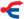关于其他名字相似的角色，请见「小云 (消歧义)」。

—— 小云，《战声渐近》，第210页

Littlecloud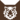虎族 (Q645)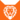狮族 (Q646)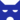影族 武士影族 学徒影族 首席巫医
（继任自奔鼻 (Q2503)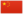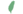小小 Littlekit  小微
 小爪 Littlepaw  小爪、微掌
 小云 Littlecloud  小雲

Q3318：影族公猫，自《呼唤野性》登场的角色

## 细节

### 外貌

• 他是一只身形极小:178且削瘦、:33:404蓝色眼睛:64的棕色虎斑公猫。:89他有一条浅棕色的尾巴:146和棕色的鼻子。:273

### 作者声明

• 维琪认为小云并没有在生病时爱上炭毛，因为她比他年长许多，但他一直很喜欢她，将她视为大姐姐。

### 勘误

• 他曾被错误地描述为灰色虎斑猫。:58

### 翻译

• 中文版的《终极指南》的〈奔鼻和小云篇〉中误称炭心是救了小云的猫，实际上应为炭毛。:84

## 登场书目

• 呼唤野性 (Q57)[配角][首次登场🐱]
• 寒冰烈火 (Q59)[猫物闲角]
• 疑云重重 (Q60)[猫物闲角]
• 风起云涌 (Q65)[配角]
• 险路惊魂 (Q66)[配角]
• 力挽狂澜 (Q67)[猫物闲角]
• 午夜追踪 (Q139)[配角]
• 新月危机 (Q140)[配角]
• 重现家园 (Q141)[配角]
• 星光指路 (Q142)[配角]
• 黄昏战争 (Q143)[配角]
• 日落和平 (Q144)[配角]
• 预视力量 (Q152)[配角]
• 暗河汹涌 (Q153)[配角]
• 驱逐之战 (Q154)[配角]
• 天蚀遮月 (Q155)[配角]
• 暗夜长影 (Q156)[配角]
• 拂晓之光 (Q157)[配角]
• 第四学徒 (Q158)[配角]
• 战声渐近 (Q159)[配角]
• 暗夜密语 (Q160)[配角]
• 月光印记 (Q161)[对话提及]
• 武士归来 (Q162)[配角]
• 群星之战 (Q163)[配角]
• 学徒探索 (Q170)[配角]
• 雷影交加 (Q171)[配角][死亡时刻✝]
• 火星的探索 (Q176)[猫物闲角]
• 黄牙的秘密 (Q180)[配角]
• 黑莓星的风暴 (Q182)[配角]
• 虎心的阴影 (Q185)[配角]
• 鸦羽的拷问 (Q186)[配角]
• 松鼠飞的希冀 (Q3561)[配角]
• 灰条的誓言 (Q4057)[猫物闲角]
• 豹星的荣光 (Q4426)[配角]
• 一星的告解 (Q4826)[配角]
• 冬青叶的故事 (Q187)[猫物闲角]
• 雾星的征兆 (Q188)[猫物闲角]
• 虎掌的愤怒 (Q190)[配角]
• 叶池的祈愿 (Q191)[猫物闲角]
• 鸽翅的沉默 (Q192)[配角]
• 褐皮的族群 (Q3566)[对话提及]
• 蛾翅的秘密 (Q3628)[配角]
• 黑脚的审判 (Q4336)[配角]
• 逐出影族 (Q4769)[配角]
• 族群的秘密 (Q199)[对话提及][追溯修订]
• 族群的猫 (Q200)[配角]
• 终极指南 (Q204)[配角]

• 巫医的天职 （提及）
• 抉择的问题 （隐射）
• 无脑的恶霸 （隐射）
• ## 脚注

1. 正体中文版中，Brownpaw被错误地译为“枯掌”。

## 参考文献

这篇文章基于CC BY-SA 3.0许可使用了猫武士维基（英语）Littlecloud一文中的部分内容。
1. 《雷影交加》第一章 (Q1580)
2. 《黄牙的秘密》第三十四章 (Q1853)
3. 《荒野新生》（繁体中文）.晨星出版社
4. 寒冰烈火 (Q59)
5. 午夜追踪
6. 鸽翅的沉默
7. 虎心的阴影
8. 终极指南
9. 风起云涌
10. 呼唤野性
11. 暗夜密语
12. Vicky Holmes (2016-07-15). 站内截图. Facebook . 原页面 归档于 2018-04-18. “Hello Mikayla, good questions! I think Runningwind was the father of Fern and Ash. You will find out the names of Cherry and Sharp's kits in the new series! And I don't think Littlecloud was ever in love with Cinderpelt because she was much older than him, but he was always very fond of her, like an older sister. Vicky x”
13. 虎掌的愤怒
14. 终极指南》，简体中文